Function Repository Resource:

# FoxHToMeijerG

Express FoxH in terms of MeijerG whenever applicable

Contributed by: Jan Mangaldan
 ResourceFunction["FoxHToMeijerG"][expr] converts FoxH expressions in expr to a form involving MeijerG. ResourceFunction["FoxHToMeijerG"][expr,f] wraps f around any MeijerG head in the result.

## Details

The Fox H-function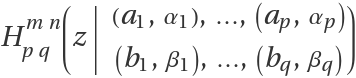can be converted to an equivalent Meijer G-function representation whenever the parameters αj and βj are rational numbers.
If any of the αj and βj are nonpositive, the function is first converted to an equivalent form where the αj and βj are positive.

## Examples

### Basic Examples (2)

A Fox H function:

 In:=Out=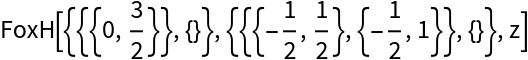Convert to an equivalent form involving Meijer G functions:

 In:=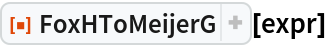Out=### Scope (4)

FoxHToMeijerG works on inactivated FoxH expressions:

 In:=Out=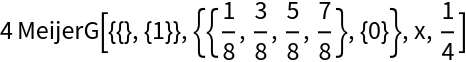Convert a Fox H expression with nonpositive αj and βj parameters:

 In:=Out=The following automatically evaluates to a form that is free from Meijer G functions, due to auto-evaluation of MeijerG:

 In:=Out=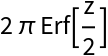Wrap the MeijerG heads in Inactive to prevent evaluation:

 In:=Out=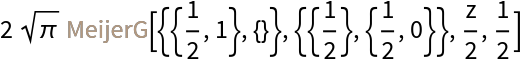Use FoxHToMeijerG on the result of FoxHReduce:

 In:=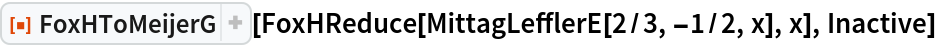Out=### Properties and Relations (2)

Use FoxHToMeijerG along with the resource function MeijerGToHypergeometricPFQ to get expressions in terms of hypergeometric functions:

 In:=Out=Activate and simplify the result:

 In:=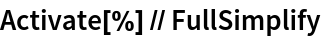Out=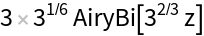### Possible Issues (1)

Fox H expressions with irrational parameters are left unchanged:

 In:=Out=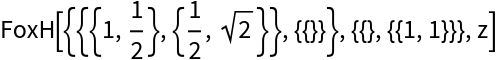## Version History

• 1.0.1 – 28 March 2022
• 1.0.0 – 04 October 2021